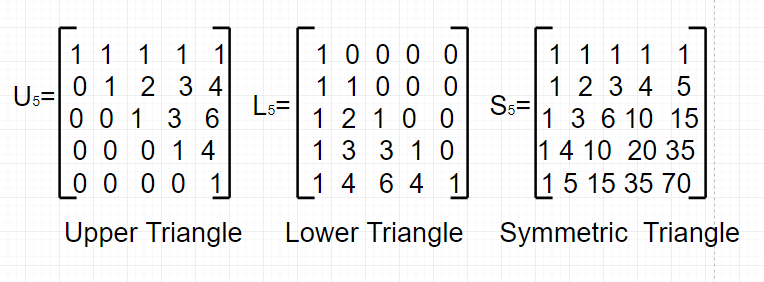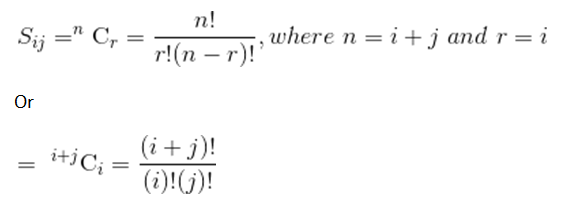# Pascal Matrix

In mathematics, particularly in matrix theory and combinatorics, the Pascal Matrix is an infinite matrix containing the binomial coefficients as its elements. There are three ways to achieve this: as either an upper-triangular matrix, a lower-triangular matrix, or a symmetric matrix. The 5 x 5 truncations of these are shown below:The elements of the symmetric Pascal Matrix are the binomial coefficient, i.eGiven a positive integer n. The task is to print the Symmetric Pascal Matrix of size n x n.
Examples:

```Input : n = 5
Output :
1 1 1 1 1
1 2 3 4 5
1 3 6 10 15
1 4 10 20 35
1 5 15 35 70
```

## Recommended: Please try your approach on {IDE} first, before moving on to the solution.

Below is the code to implement n x n symmetric pascal matrix:

## C++

 `// CPP Program to print symmetric pascal matrix. ` `#include ` `using` `namespace` `std; ` ` `  `// Print Pascal Matrix ` `void` `printpascalmatrix(``int` `n) ` `{ ` `    ``int` `C[2 * n + 1][2 * n + 1] = { 0 }; ` ` `  `    ``// Calculate value of Binomial Coefficient in ` `    ``// bottom up manner ` `    ``for` `(``int` `i = 0; i <= 2 * n; i++) { ` `        ``for` `(``int` `j = 0; j <= min(i, 2 * n); j++) { ` ` `  `            ``// Base Cases ` `            ``if` `(j == 0 || j == i) ` `                ``C[i][j] = 1; ` ` `  `            ``// Calculate value using previously ` `            ``// stored values ` `            ``else` `                ``C[i][j] = C[i - 1][j - 1] + C[i - 1][j]; ` `        ``} ` `    ``} ` ` `  `    ``// Printing the pascal matrix ` `    ``for` `(``int` `i = 0; i < n; i++) { ` `        ``for` `(``int` `j = 0; j < n; j++) ` `            ``cout << C[i + j][i] << ``" "``; ` ` `  `        ``cout << endl; ` `    ``} ` `} ` ` `  `// Driven Program ` `int` `main() ` `{ ` `    ``int` `n = 5; ` `    ``printpascalmatrix(n); ` `    ``return` `0; ` `} `

## Java

 `// java Program to print  ` `// symmetric pascal matrix. ` `import` `java.io.*; ` ` `  `class` `GFG  ` `{ ` `    ``// Print Pascal Matrix ` `    ``static` `void` `printpascalmatrix(``int` `n) ` `    ``{ ` `        ``int` `C[][] = ``new` `int``[``2` `* n + ``1``][``2` `* n + ``1``]; ` `     `  `        ``// Calculate value of Binomial Coefficient in ` `        ``// bottom up manner ` `        ``for` `(``int` `i = ``0``; i <= ``2` `* n; i++)  ` `        ``{ ` `            ``for` `(``int` `j = ``0``; j <= Math.min(i, ``2` `* n); j++)  ` `            ``{ ` `                ``// Base Cases ` `                ``if` `(j == ``0` `|| j == i) ` `                    ``C[i][j] = ``1``; ` `     `  `                ``// Calculate value using previously ` `                ``// stored values ` `                ``else` `                    ``C[i][j] = C[i - ``1``][j - ``1``]  ` `                              ``+ C[i - ``1``][j]; ` `            ``} ` `        ``} ` `     `  `        ``// Printing the pascal matrix ` `        ``for` `(``int` `i = ``0``; i < n; i++) ` `        ``{ ` `            ``for` `(``int` `j = ``0``; j < n; j++) ` `                ``System.out.print ( C[i + j][i] +``" "``); ` `                ``System.out.println(); ` `         `  `        ``} ` `    ``} ` `     `  `    ``// Driven Program ` `    ``public` `static` `void` `main (String[] args)  ` `    ``{ ` `        ``int` `n = ``5``; ` `        ``printpascalmatrix(n); ` `     `  `    ``} ` `} ` ` `  `// This code is contributed by vt_m. `

## Python3

 `# Python3 Program to print  ` `# symmetric pascal matrix. ` ` `  `# Print Pascal Matrix ` `def` `printpascalmatrix(n): ` `    ``C ``=` `[[``0` `for` `x ``in` `range``(``2` `*` `n ``+` `1``)]  ` `            ``for` `y ``in` `range``(``2` `*` `n ``+` `1``)]  ` `             `  `    ``# Calculate value of  ` `    ``# Binomial Coefficient ` `    ``# in ottom up manner ` `    ``for` `i ``in` `range``(``2` `*` `n ``+` `1``): ` `        ``for` `j ``in` `range``(``min``(i, ``2` `*` `n) ``+` `1``): ` `             `  `            ``# Base Cases ` `            ``if` `(j ``=``=` `0` `or` `j ``=``=` `i): ` `                ``C[i][j] ``=` `1``; ` `                 `  `            ``# Calculate value ` `            ``# using previously ` `            ``# stored values ` `            ``else``: ` `                ``C[i][j] ``=` `(C[i ``-` `1``][j ``-` `1``] ``+`  `                           ``C[i ``-` `1``][j]); ` `     `  `    ``# Printing the ` `    ``# pascal matrix ` `    ``for` `i ``in` `range``(n): ` `        ``for` `j ``in` `range``(n): ` `            ``print``(C[i ``+` `j][i],  ` `                   ``end ``=` `" "``); ` `        ``print``(); ` `     `  `# Driver Code ` `n ``=` `5``; ` `printpascalmatrix(n); ` ` `  `# This code is contributed by mits `

## C#

 `// C# program to print ` `// symmetric pascal matrix. ` `using` `System; ` ` `  `class` `GFG { ` `     `  `    ``// Print Pascal Matrix ` `    ``static` `void` `printpascalmatrix(``int` `n) ` `    ``{ ` `        ``int``[, ] C = ``new` `int``[2 * n + 1, 2 * n + 1]; ` ` `  `        ``// Calculate value of Binomial Coefficient  ` `        ``// in bottom up manner ` `        ``for` `(``int` `i = 0; i <= 2 * n; i++) { ` `             `  `            ``for` `(``int` `j = 0; j <= Math.Min(i, 2 * n); j++) { ` `                 `  `                ``// Base Cases ` `                ``if` `(j == 0 || j == i) ` `                    ``C[i, j] = 1; ` ` `  `                ``// Calculate value using previously ` `                ``// stored values ` `                ``else` `                    ``C[i, j] = C[i - 1, j - 1] ` `                            ``+ C[i - 1, j]; ` `            ``} ` `        ``} ` ` `  `        ``// Printing the pascal matrix ` `        ``for` `(``int` `i = 0; i < n; i++) { ` `            ``for` `(``int` `j = 0; j < n; j++) ` `                ``Console.Write(C[i + j, i] + ``" "``); ` `            ``Console.WriteLine(); ` `        ``} ` `    ``} ` ` `  `    ``// Driven Program ` `    ``public` `static` `void` `Main() ` `    ``{ ` `        ``int` `n = 5; ` `        ``printpascalmatrix(n); ` `    ``} ` `} ` ` `  `// This code is contributed by vt_m. `

## PHP

 ` `

Output:

```1 1 1 1 1
1 2 3 4 5
1 3 6 10 15
1 4 10 20 35
1 5 15 35 70
```

My Personal Notes arrow_drop_upCheck out this Author's contributed articles.

If you like GeeksforGeeks and would like to contribute, you can also write an article using contribute.geeksforgeeks.org or mail your article to contribute@geeksforgeeks.org. See your article appearing on the GeeksforGeeks main page and help other Geeks.

Please Improve this article if you find anything incorrect by clicking on the "Improve Article" button below.

Improved By : jit_t, Mithun Kumar